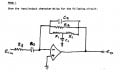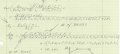# Need an Approximation of a Bode Plot

#### sailmike

Joined Nov 11, 2013
147
I need an approximation of what a bode plot would look like with 2 zeros and 3 poles for a bandpass amplifier. I know that at f = 0 no current flows at all and at f = infinity, gain goes to zero. There are no numbers because I have just R1, R2, R3, C1, C2, and C3 for this circuit. The equation is very long and messy, but there's an S^2 in the numerator and an S^3 in the denominator.

Thanks,
Mike#### MrAl

Joined Jun 17, 2014
9,779
Hi,

First, that looks like a variation on an active Twin T Notch Filter. They are capable of producing a very 'deep' notch because of the interesting way the network impedance behaves over frequency. We can do a full analysis to find out for sure later if you like, but it sounds like you may have already done something like that. Yes, the equation comes out somewhat complicated with all the components involved. Some of them are the same however in most circuits, like the capacitors or are related by a constant factor as simple as "2" or "1/2".

To approximate, you might recall that a zero causes a rise in gain with frequency while a pole causes a decrease in gain with frequency. The amount of rise for the zero matches the amount of decrease for the pole.
This means that if in frequency you start with one zero the gain increases until you reach a pole, where it then decreases at the same rate as the zero so the amplitude levels off. Then if you come to another pole the gain would decrease again and if that was all we had that would be a bandpass filter.

This is the homework section however, so you should state how you approach this problem and what you ended up with before i give out any more information on this. That's the general policy we follow here.
Make at least some attempt to figure this out, or at least what you think might workLooking at it a little more carefully, it's not a twin T notch filter obviously there is no "twin T" network, but the analysis would go much the same way. It could be a bandpass of sorts, but you might want to mention what you think it is first.

Last edited:

#### sailmike

Joined Nov 11, 2013
147
Without any values for the resistors or capacitors I'm not sure how to approximate the plot. First I set f= 0 and see that C3 makes it an open circuit, so the gain is zero. When I set f= infinity, C3 and C2 are short circuits, so the gain is again zero. I know zeros represent a gain of 20 db and poles represent a loss of 20 db. I see 3 losses of 20 db each and 2 gains of 20 db each. I don't know how to analyze the equation when there's no numbers. It's been a while since I've been in class so I need a reminder.#### MrAl

Joined Jun 17, 2014
9,779
Hi,

Oh, so this isnt really homework then? When we think of homework here we mean that given by a teacher of some sort for a student who has to do the problems so they learn how to do them, usually for the first time ever.

If this is not strictly homework then you really should have put it in another section, but i'll wait for your answer. We can work on this either way though.

So far it looks like you are on the right path. It looks like you have determined the transfer function and start to identify the basic shape of the output. That's already part of it done. I want to note though that i did not test your transfer function yet, but if you have the right transfer function and factor the denominator, one pole will already jump out at you. That will give you a starting point.

When you plot without actual numerical values to work with, you usually determine for example the pole frequencies from the values like R1*C1 and then just draw the line noting where that line started by writing the pole frequency on the 'x' axis in terms of the variables like R1 and C1, which might look like 1/(R1*C1) for example. So you print that instead of a number like "3.4" or whatever.
You can also test by using some real numbers for the values.
You also already know that you have a zero that starts the curve, then probably a complex pole that levels the curve off, then another pole that pulls the curve back down so the shape looks similar to what you have drawn, although the bandwidth could be wide or narrow, we dont know without actual values, but we still know the general shape.

So see if you can find that one pole to start with, and then see how it goes. I'll wait for your next reply before talking too much more here#### dannyf

Joined Sep 13, 2015
2,197
Just think of capacitors aa resistors whose valued change with frequency then work our the gain as a function of frequency.

#### sailmike

Joined Nov 11, 2013
147
Danny, I'm not sure what you mean. Would you mind giving me an example?

Thanks,
Mike

#### shteii01

Joined Feb 19, 2010
4,644
Danny, I'm not sure what you mean. Would you mind giving me an example?

Thanks,
Mike
Capacitor impedance is $$Z_C=\frac{1}{sC}=\frac{1}{jwC}$$.
C is value of the capacitor in Farads, it DOES NOT change.
w is the frequency in radians per second. Notice that w is in denominator. What you do is you plug different values of w into the equation. So you start at 0.001 rad/s and go to, for example, 10 million rad/s. As w increses, $$Z_C=\frac{1}{jwC}$$ becomes smaller and smaller and smaller because you keep dividing 1 by larger and larger values because w keeps increasing. That is what dannyf meant when they said that value of the capacitor in the form of "resistor" (capacitor impedance) will change with frequency.

#### MrAl

Joined Jun 17, 2014
9,779
Without any values for the resistors or capacitors I'm not sure how to approximate the plot. First I set f= 0 and see that C3 makes it an open circuit, so the gain is zero. When I set f= infinity, C3 and C2 are short circuits, so the gain is again zero. I know zeros represent a gain of 20 db and poles represent a loss of 20 db. I see 3 losses of 20 db each and 2 gains of 20 db each. I don't know how to analyze the equation when there's no numbers. It's been a while since I've been in class so I need a reminder.

View attachment 104333

Hello again,

Let me put this another way...

When you do the transfer function analytically, you end up with R's and C's and s's with possibly some numbers, and the denominator will be third degree. If you find the right transfer function however, you will find that you can factor it into a second degree times a first degree expression.
So the denominator will be in the form:
(A*s+1)*(B*s^2+C*s+D)

where A, B, C, and D are all constants which depend on the circuit topology, and i will presume you get the right transfer function so you get the right constants for these four.

The first thing you can do because of the (A*s+1) factor is you can divide the numerator and denominator by 'A', so we get for the denominator now:
(s+1/A)*(B*s^2+C*s+D)

See how simple that was so far? But remember that A gets divided into the numerator also, so if we call the original numerator N for now it would then become:
new N=N/A

So effectively all we have done so far is divide top and bottom by A, and writing it out a little it looks like this:
(N/A)/(D/A)
where D is the denominator above.

Now the form we ended up with for D was:
(s+1/A)*(B*s^2+C*s+D)

and what this means is that one of the poles is 1/A.

Assuming we start with a pole, in the graph we would just write 1/A near the start of the x axis (which is the frequency axis) and then draw a diagonal line starting from that point on the x axis downwards and to the right, so it would look like a big backslash: "\"
so the graph so far would something like this:
Code:
______(1/A)
\

where the horizontal line is the x axis. That 1/A indicates where the pole starts out (the break frequency).

So instead of writing a number, we write a fraction that comes from the transfer function. For this transfer function A would be R3*C3 so we would have as one pole at w=1/(R3*C3).

Next we have the quadratic (B*s^2+C*s+D). There will be two poles from this, and to find them both we have to factor that quadratic.
To factor, we could use the quadratic solution:
r1=-b+sqrt(b^2+4*a*c)/(2*a)
r2=-b-sqrt(b^2+4*a*c)/(2*a)
where a=B, b=C, and c=D in the denominator quadratic.

The factorization is then:
a*(s-r1)*(s-r2)

Note the roots r1 and r2 may be more complicated then we would like, so we may just stick with r1 and r2 and make a side note as to what they actually are in terms of the denominator quadratic:
r1=such and such 1
r2=such and such 2
and:
a=B

Ok, so we have the denominator part now:
a*(s-r1)*(s-r2)

and let's make the r1 and r2 added by defining:
R1=-r1
R2=-r2

now we can write the denominator part as:
a*(s+R1)*(s+R2)

and thus we have the two poles, R1 and R2, and 'a' becomes part of the numerator constant.

So there you have it, the three poles extracted from the denominator. To do the numerator you would follow a similar procedure.
You'll have to figure out the order of the poles and zeros, such as p1<z1<z2<p2<p3 or something like thatfrom the shape of the response.

EDIT: Original post showed the pole as increasing the gain but it really decreases the gain. This has been fixed.

Last edited: